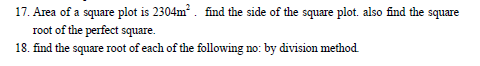# SCC Education

## Square and Square Roots important questionsSquare and Square Roots
1-Which of the following numbers are perfect squares?
(a)3364 (b)4489 (c)4358
2-Using the property of squares , find the value of the following.
(a)242 - 232 (b)502 – 492 (c)1052 – 1042

(a)1+3+5+7+9
(b)1+3+5+7+9+11+13+15_17+19+21+23+
4-Find the square root of the following by prime factorisation method.
(a)1024 (b)8836 (c)8464
5-Find the smallest number by which 2475 must be multiplied to get a perfect square .
6-4096 soldiers are arranged in an auditorium in such a manner that there are as many soldiers in a row as there are rows in the auditorium.How many rows are there in the auditorium?
7-Find the least perfect square exactly divisible by each one of the numbers 4, 5, 10.
8-Find the least square number exactly divisible by each one of the numbers 6, 9, 10, 15 and 20 .
9-Find the least number which must be added to 4215 to make it a perfect square.
10-Find the lest number which should be subtracted from 984 to make it a perfect square .Also find the square root of this perfect square.
11-The area of the square field is 8464m2. A man takes 3 rounds of this field. Find the distance covered by him.
12-Find the square root of the following decimal numbers.
(a)72.25 (b)86.49 (c)75.69 (d)9.61 (e)0.16
14-Express 81 as the sum of 9 odd numbers.
15-How many numbers lie between the squares of the following numbers?
(a)15 and 16 (b)56 and 57
16-Express the following as the sum of two consecutive integers.
(a)212 (b)132 (c)192Mathematics for class 8 ..........

Formula-sheet
It will help you to solve the questions of mensuration like area ,volume ,Surface area etc.

Rule of divisibility

It will help you to under stand rule of divide .how to check a number is divisible or not.

A fraction is known as a rational number and written in the form of p/q where p and q are  integers and q ≠ 0. The lower number ‘q’ is known as denominator and the upper  number ‘p’ is known as numerator. Type of Fractions Proper Fraction: The fraction in which numerator is less the denominator is called a  proper fraction.

Angles An angle is formed when two lines meet. The size of the angle measures the amount of space between the lines. In the diagram the lines ba and bc are called the ‘arms’ of the angle, and the point ‘b’ at which they meet is called the ‘vertex’ of the angle.

Properties-of-parallel-lines

Parallelograms properties

Volume-and-area-of-cone-and-pyramid

Profit-and-loss-questions

Compound-interest

Volumes-surface-and-area

Percentage-and-its-application

Test paper Algebra

Test paper redicals

Rational number

Profit and loss

Work problem

Power questions
formula-sheet

properties-of-parallel-lines

parallelograms properties

volume-and-area-of-cone-and-pyramid

profit-and-loss-questions

compound-interest

volumes-surface-and-area

percentage-and-its-application